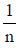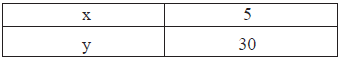Courses

# Direct And Inverse Proportions - MCQ

## 10 Questions MCQ Test Class 8 Mathematics by VP Classes | Direct And Inverse Proportions - MCQ

Description
This mock test of Direct And Inverse Proportions - MCQ for Class 8 helps you for every Class 8 entrance exam. This contains 10 Multiple Choice Questions for Class 8 Direct And Inverse Proportions - MCQ (mcq) to study with solutions a complete question bank. The solved questions answers in this Direct And Inverse Proportions - MCQ quiz give you a good mix of easy questions and tough questions. Class 8 students definitely take this Direct And Inverse Proportions - MCQ exercise for a better result in the exam. You can find other Direct And Inverse Proportions - MCQ extra questions, long questions & short questions for Class 8 on EduRev as well by searching above.
QUESTION: 1

Solution:
QUESTION: 2

Solution:
QUESTION: 3

### If ‘A’ can finish a work in‘n’ days then part of work finished in 1 day is:

Solution:
QUESTION: 4

If amount of work completed by ‘A’ in one day isthen the whole work will be finished by ‘A’ is:

Solution:
QUESTION: 5

If an increase in one quantity brings about a corresponding decrease in the other and ice versa,then the two quantities vary:

Solution:
QUESTION: 6

“If speed is more than time to cover a fixed distance would be less”. This is a case of :

Solution:
QUESTION: 7

Which of the following is a case of inverse variation?

Solution:
QUESTION: 8

Which of the following is not a case of direct variation?

Solution:
QUESTION: 9

Which of the following is case of direct variation:

Solution:
QUESTION: 10

If x and y vary inversely. Then using the following table?The value of x for y = 10 is

Solution: